## How does Tandem Repeats Finder work?

 • Probabilistic Model of Tandem Repeats • Program Outline • Detection Component • Statistical Criteria • Sum of Heads Distribution • Random Walk Distribution • Apparent Size Distribution • Waiting Time Distribution • Analysis Component • Program Parameters • Redundancy

Probabilistic Model of Tandem Repeats

We model alignment of two tandem copies of a pattern of length n by a sequence of n independent Bernoulli trials (coin-tosses). The probability of success, P(Heads), which we also callor matching probability, represents the average percent identity between the copies. Each head in the Bernoulli sequence is interpreted as a match between aligned nucleotides. Each tail is a mismatch, insertion or deletion. A second probability,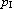or indel probability, specifies the average percentage of insertions and deletions between the copies. Figure 1 illustrates the underlying idea for the model.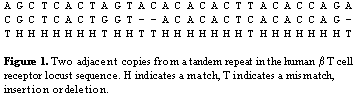We are interested in the distribution of Bernoulli sequences and the properties of alignments that they represent when dealing with a specific pair (,), for example, (= .80,= .10). Note that these conservation parameters serves as a type of extremal bound, i.e., as a quantitative description of the most divergent copies we hope to detect.

Program Outline

The program has detection and analysis components. The detection component uses a set of statistically based criteria to find candidate tandem repeats. The analysis component attempts to produce an alignment for each candidate and if successful gathers a number of statistics about the alignment (percent identity, percent indels) and the nucleotide sequence (composition, entropy measure).

Detection Component

We assume that adjacent copies of any pattern will contain some matching characters in corresponding positions. Just how many matches and how the distance between those matches should vary depend on the fixed values ofand. We use statistical criteria to answer these questions.

The algorithm looks for matching nucleotides separated by a common distance d, which is not specified in advance. For reasons of efficiency it looks for runs of k matches, which we call k-tuple matches. A k-tuple is a window of k consecutive characters from the nucleotide sequence. Matching k-tuples are two windows with identical contents and if aligned in the Bernoulli model would produce a run of k heads. Because we limit ourselves to k-tuple matches, we will not detect all matching characters. For example, if k=6 and two windows contain TCATGT and TCTTGT we will not know that there are 5 matching characters because the window contents are not identical. Put in terms of the Bernoulli model, the aligned windows would be represented by the sequence HHTHHH which is not a run of 6 heads.

The basic operation of the detection component is illustrated in the figure below. Let S be a nucleotide sequence. We select a small integer k, for the tuple or window size (k=5 for example) and keep a list of all possible k-length strings (there are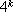for the DNA alphabet {A,C,G,T}) which we call the probes. By sliding the window across the sequence, we determine the probe at each position i in S. For each probe p, we maintain a history list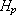of the positions at which p occurs.

When a position i is added to, we scanfor all earlier occurrences of p. Let one earlier occurrence be at j. Since i and j are the indices of matching k-tuples, the distance d=i-j is a possible pattern size for a tandem repeat. For the criteria tests, we need information about other k -tuple matches at the same distance d where the leading tuple occurs in the sequence between j and i. A distance list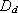stores this information. It can be thought of as a sliding window of length d which keeps track of the positions of matches and their total.

Listis updated every time a match at distance d is detected. Position i of the match is stored on the list and the total is increased. The right end of the window is set to i and matches that occurred before j=i-d are dropped from the list and subtracted from the total. Lists for other nearby distances are also updated at this time (see Random Walk Distribution ), but only to reset their right ends to i and remove matches that have been passed by the advancing windows. Information in the updated distance lists is used for the sum-of-heads and apparent-size criteria tests. If both tests are successful, the program moves on to the analysis component.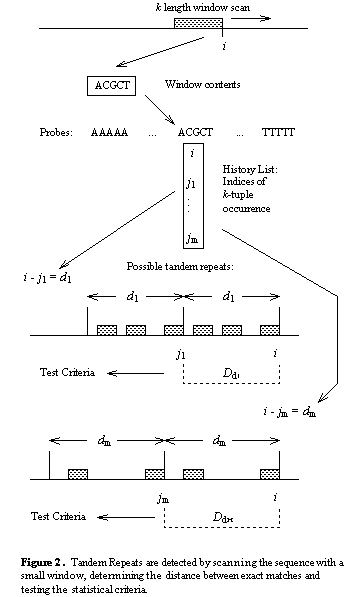Statistical Criteria

The statistical criteria are based on runs of heads in Bernoulli sequences, corresponding to matches detected with the k-tuples and stored in the distance lists. The criteria are based on four distributions which depend upon 1) the pattern length, d, 2) the matching probability,, 3) the indel probability,, and 4) the tuple size, k. For each distribution, we either calculate it with a formula or estimate it using simulation. Then, we select a cutoff value that serves as our criterion.

This distribution indicates how many matches are required for a specific distance or pattern length. Let the random variable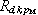= the total number of heads in head runs of length k or longer in an iid Bernoulli sequence of length d with success probability. The distribution ofis well approximated by the normal distribution and its exact mean and variance can be calculated in constant time. For the sum-of-heads criterion, we use the normal distribution to determine the largest number, x, such that 95% of the timex. For example, if= .75, k = 5 and d = 100, then the criterion is 26. Put another way, if a pattern has length 100 and aligned copies are expected to match in 75 positions, then by counting only matches that fill a window of length 5, we expect to count at least 26 matches 95% of the time.

Random Walk Distribution

This distribution describes how distances between matches may vary due to indels. Because indels change the distance between matching k -tuples (figure below), there will be situations where the pattern has size d, yet the distance between matching k-tuples is d±1, d±2, etc. In order to test the sum-of-heads criterion, we count the matches in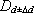, for Δd = 0,1,...,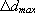for some . In our model, indels are single nucleotide events occurring with probability. Insertions and deletions are considered equally likely and we treat the distance change as a problem of random walks. Let the random variable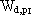= the maximum displacement from the origin of a one dimensional random walk with expected number of steps equal to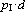. It can be shown that 95% of the time the random walk ranges between ±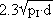. We set= || . For example if= 0.1 and d = 100, then= 7.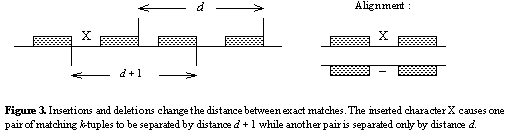Apparent Size Distribution

This distribution is used to distinguish between tandem repeats and non-tandem direct repeats (figure below). For tandem repeats, the leading tuple in matching k-tuples will be distributed throughout the interval from j to i, whereas for non-tandem repeats, they should be concentrated on the right side of the interval near i. Let the random variable= The distance between the first and last run of k heads in an iid Bernoulli sequence of length d with success probability.is the apparent size of the repeat when using k-tuples to find the matches and will usually be shorter than the pattern size d. We estimate the distribution ofby simulation because we make it conditional on first meeting the sum-of-heads criterion. For given d,k, and, random Bernoulli sequences are generated using. For every sequence that meets or exceeds the sum-of-heads criteria, the distance between the first and last run of heads of length k or larger is recorded. From the distribution, we determine the maximum number y such that 95% of the timeis greater than y . We use y as our apparent size criterion. For example, if= .75, k = 5 and d = 100, then the criterion is 56. In order to test the apparent- size criterion, we compute the distance between the first and last tuple on list. If the distance between the tuples is smaller than the criterion, we assume the repeat is not tandem or that we have not yet seen enough of it to be convinced.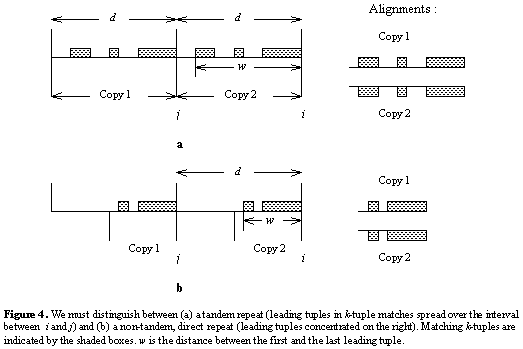Waiting Time Distribution

This distribution is used to pick tuple sizes. Tuple size has a significant inverse effect on the running time of the program because increasing tuple size causes an exponential decrease in the expected number of tuple matches. If the nucleotides occur with equal frequency, then increasing the tuple size by Δk increases the average distance between randomly matching tuples by a factor of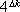. If k=5, the average distance between random matches is approximately 1Kb, but if k=7, the average distance is approximately 16Kb. Thus, by using a larger tuple size, we keep the history lists short. On the other hand, increasing the tuple size decreases the chance of noticing approximate copies because they may not contain a long, unbroken run of matches. Let the random variable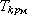= the number of iid Bernoulli trials with success probabilityuntil the first occurrence of a run of k successes.follows the geometric distribution of order k. If we let p =and q=1-p then the exact probabilityfor x ≥ 0 is given by the recursive formula :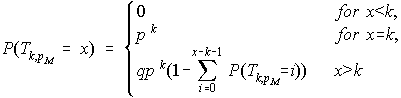For example, if= .75 and k = 5 then we need at least 31 trials (coin-tosses) to have a 95% chance of seeing a run of 5 heads. For patterns smaller than 31 characters, we need to use a smaller k-tuple. The waiting time distribution allows us to balance the running time and sensitivity of our algorithm by picking a set of tuple sizes, each applying to a different range of pattern sizes. The program processes the sequence once, simultaneously checking these different tuple sizes. We require that the smallest pattern for tuple size k have a sum-of-heads criterion of at least k+1. The table below shows the range of tuple sizes and the corresponding pattern sizes currently used by the program.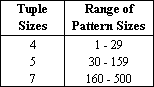Analysis Component

If the information in the distance list passes the criteria tests, a candidate pattern consisting of positions j+1...i, is selected from the nucleotide sequence and aligned with the surrounding sequence using wraparound dynamic programming (WDP). If at least two copies of the pattern are aligned with the sequence, the tandem repeat is reported. Several implementation details of the analysis component are described below.

• Multiple Reporting of Repeat at Different Pattern Sizes :

When a single tandem repeat contains many copies, several pattern sizes are possible. For example, if the basic pattern size is 26, then the repeat may be reported at sizes 26, 52, 78, etc. We limit this redundancy in the output to, at most, three pattern sizes. Note that we do not automatically limit the output to the smallest period size because a much better alignment may come from a larger size.

• Narrow Band Alignment :

Alignments are the program's most time intensive calculations. To decrease running time, we limit WDP calculations to a narrow diagonal band in the alignment matrix for patterns larger than 20 characters. In accordance with the random walk results, the band radius is. The band is periodically recentered around a run of matches in the current best alignment.

• Consensus Pattern and Period Size :

An initial candidate pattern P is drawn from the sequence, but this is usually not the best pattern to align with the tandem repeat. To improve the alignment, we determine a consensus pattern by majority rule from the alignment of the copies with P. The consensus is used to realign the sequence and this final alignment is reported in the output. Period size is defined as the most common matching distance between corresponding characters in the alignment and may not be identical to consensus size.

Program Parameters

Input to the program consists of a sequence file and the following parameters ( allowable parameters values are shown in the main window ) :

1) Alignment weights for match, mismatch and indels. These parameters are for Smith-Waterman style local alignment using wraparound dynamic programming. Lower weights allow alignments with more mismatch and indels. Match weight is +2 in all options here. Mismatch and indel weights ( interpreted as negative numbers are either 3, 5, or 7. 3 is more permissive and 7 is less permissive of these types of alignments choices.

2) Matching probabilityand indel probability.= .80 and= .10 by default and can only be modified in the console version of the program.

3) A maximum period size for patterns to report. Period size is the program's best guess at the pattern size of the tandem repeat. The program will find all repeats with period size between 1 and 2000, but the output table can be limited to some other range.

4) A minimum alignment score to report repeat. The alignment of a tandem repeat must meet or exceed the alignment score to be reported.

Redundancy

Tandem Repeats Finder finds repeats for period sizes in the range from 1 to 2000 nucleotides. If a repeat contains many copies, then the same repeat will be detected at various period sizes. This is restricted to the three best scoring period sizes. For example if a tandem repeat has period size 3 and contains 30 copies, then it will most likely also be detected at size 6 with 15 copies and size 9 with 7.5 copies. Also, the same period size may be detected more than once with different scores and slightly different indices.Last revised October 20, 2022 Send any questions or comments to: Gary Benson